Subsections

# 3.2 DLList: A Doubly-Linked List

A DLList (doubly-linked list) is very similar to an SLList except that each node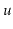in a DLList has references to both the node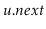that follows it and the node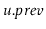that precedes it.

When implementing an SLList, we saw that there were always several special cases to worry about. For example, removing the last element from an SLList or adding an element to an empty SLList requires care to ensure that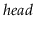andare correctly updated. In a DLList, the number of these special cases increases considerably. Perhaps the cleanest way to take care of all these special cases in a DLList is to introduce anode. This is a node that does not contain any data, but acts as a placeholder so that there are no special nodes; every node has both a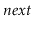and a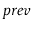, withacting as the node that follows the last node in the list and that precedes the first node in the list. In this way, the nodes of the list are (doubly-)linked into a cycle, as illustrated in Figure 3.2.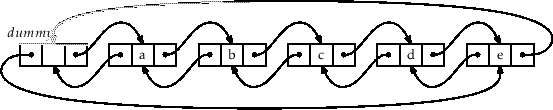Finding the node with a particular index in a DLList is easy; we can either start at the head of the list () and work forward, or start at the tail of the list () and work backward. This allows us to reach the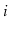th node intime:Theand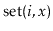operations are now also easy. We first find theth node and then get or set its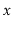value: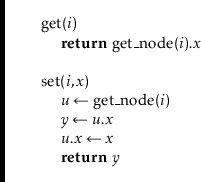The running time of these operations is dominated by the time it takes to find theth node, and is therefore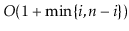.

## 3.2.1 Adding and Removing

If we have a reference to a nodein a DLList and we want to insert a nodebefore, then this is just a matter of setting,, and then adjusting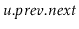and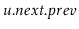. (See Figure 3.3.) Thanks to the dummy node, there is no need to worry about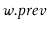or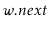not existing.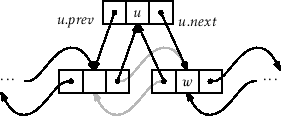Now, the list operationis trivial to implement. We find theth node in the DLList and insert a new nodethat containsjust before it.The only non-constant part of the running time ofis the time it takes to find theth node (using). Thus,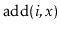runs intime.

Removing a nodefrom a DLList is easy. We only need to adjust pointers atandso that they skip over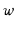. Again, the use of the dummy node eliminates the need to consider any special cases: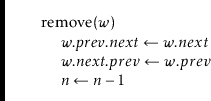Now the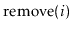operation is trivial. We find the node with indexand remove it:Again, the only expensive part of this operation is finding theth node using, soruns intime.

## 3.2.2 Summary

The following theorem summarizes the performance of a DLList:

Theorem 3..2   A DLList implements the List interface. In this implementation, the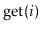,,andoperations run intime per operation.

It is worth noting that, if we ignore the cost of theoperation, then all operations on a DLList take constant time. Thus, the only expensive part of operations on a DLList is finding the relevant node. Once we have the relevant node, adding, removing, or accessing the data at that node takes only constant time.

This is in sharp contrast to the array-based List implementations of Chapter 2; in those implementations, the relevant array item can be found in constant time. However, addition or removal requires shifting elements in the array and, in general, takes non-constant time.

For this reason, linked list structures are well-suited to applications where references to list nodes can be obtained through external means.

opendatastructures.org